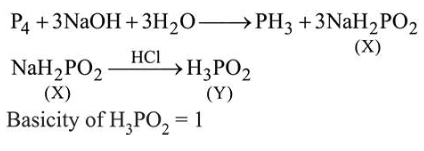# White phosphorus on reaction with concentrated

Question:

White phosphorus on reaction with concentrated $\mathrm{NaOH}$ solution in an inert atmosphere of $\mathrm{CO}_{2}$ gives phosphine and compound $(X) .(X)$ on acidification with $\mathrm{HCl}$ gives compound $(Y)$. The basicity of compound $(Y)$ is:

1. 2

2. 1

3. 4

4. 3

Correct Option: , 2

Solution: